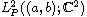Electron. J. Differential Equations, Vol. 2019 (2019), No. 02, pp. 1-14.

### Spectral analysis of singular Hamiltonian systems with an eigenparameter in the boundary condition Bilender P. Allahverdiev

Abstract:
In this article we study a non-self-adjoint eigenparameter dependent singular differential 1D Hamiltonian system with the singular end points a and b in the Hilbert spaceand we consider that this 1D Hamiltonian system is in the limit-circle cases at a and b. For this purpose we use the maximal dissipative operator associated with the considered problem whose spectral analysis is sufficient for boundary value problem. Self-adjoint dilation theory of Sz.-Nagy-Foias developed for the dissipative operators is used. Moreover we construct incoming and outgoing spectral representations of the self-adjoint dilation. This representations allows us to determine the scattering matrix. Therefore a functional model of the dissipative operator is constructed. Moreover, a functional model of the dissipative operator is constructed and its characteristic function in terms of solutions of the corresponding Hamiltonian system is described. Therefore using the obtained results for the characteristic function theory, theorems on completeness of the system of eigenvectors and associated vectors of the dissipative operator and Hamiltonian boundary value problem have been proved.

Submitted February 15, 2018. Published January 2, 2019.
Math Subject Classifications: 34B08, 34A30, 34L10, 34L25, 34L40, 47A20, 47A40.
Key Words: Hamiltonian system; limit-circle; dissipative operator; spectral parameter in the boundary; self-adjoint dilation, scattering matrix; functional model; characteristic function.

Show me the PDF file (280 KB), TEX file for this article.Bilender P. Allahverdiev Department of Mathematics Suleyman Demirel University 32260 Isparta, Turkey email: bilenderpasaoglu@sdu.edu.tr